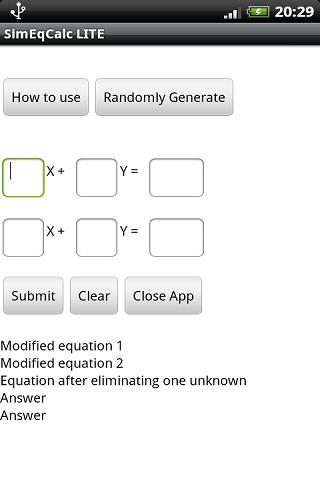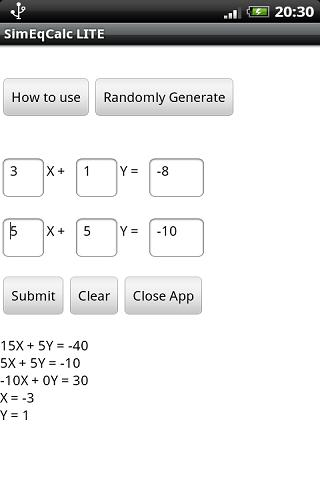# Simultaneous Equation CalcCalculator that solves simultaneous equations in the format shown below:
2x+3y=7
4x+2y=10

This will give not only the unknown X and Y variable values, but also the equation calculations used to get them. The app can randomly generate working pairs of linear equations that give integer answers.

apps2sd available

### App Specification

• App Name: Simultaneous Equation Calc
• Rating:
• Apk: appinventor.ai_brandonheatbeyondthegrave.SimultaneousEquationsCalculator
• Cost: US\$0.79
• Developer: http://www.daveplumbzero.co.uk
• Market: market://search?q=pname:appinventor.ai_brandonheatbeyondthegrave.SimultaneousEquationsCalculator